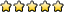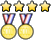Astronomy C

Test your knowledge of various Science Olympiad events.
Skink
Exalted MemberPosts: 947
Joined: February 8th, 2009, 12:23 pm
Division: C
State: IL

Re: Astronomy C

The apparent position of star A from Earth is measured in March, and then again 6 months later in September. If the apparent position of star A changed by 0.036 arcseconds, what is the approximate distance from the sun to star A in lightyears?
d (in parsecs) = 1/p (in arcseconds).  p = 0.036 arcseconds. Substituting this in, we get ~27.77 parsecs. Multiply by 3.26 to get LY, giving us [b]90.55 LY[/b]. Do we need to worry about sig figs in astro?
Mhm, C Division has a blanket sig figs policy. Whether it's enforced is another story.

ModeratorPosts: 473
Joined: December 6th, 2013, 1:56 pm
State: TX
Location: Austin, Texas

Re: Astronomy C

The apparent position of star A from Earth is measured in March, and then again 6 months later in September. If the apparent position of star A changed by 0.036 arcseconds, what is the approximate distance from the sun to star A in lightyears?
d (in parsecs) = 1/p (in arcseconds).  p = 0.036 arcseconds. Substituting this in, we get ~27.77 parsecs. Multiply by 3.26 to get LY, giving us [b]90.55 LY[/b]. Do we need to worry about sig figs in astro?
Mhm, C Division has a blanket sig figs policy. Whether it's enforced is another story.
Thanks Skink. Guess my answer should have been this:
d (in parsecs) = 1/p (in arcseconds).  p = 0.036 arcseconds. Substituting this in, we get ~27.77 parsecs, [b]but 0.036 has 2 sig figs so 28[/b].Multiply by 3.26 to get LY and round to 2 sig figs, giving us [b]91 LY[/b].
University of Texas at Austin '22
Seven Lakes High School '18
Beckendorff Junior High '14

finagle29
Exalted MemberPosts: 51
Joined: January 28th, 2013, 11:37 am
State: PA
Location: Caltech

Re: Astronomy C

Suppose a person is looking at two stars, A and B, and perceives A to be 10 times brighter than B. The product of the magnitudes of the stars is 9, and both magnitudes are positive. What are the magnitudes of both stars?
A: 2, B: 4.5
From the equation for apparent magnitude $m_a - m_b = -2.5 \log_{10}\left(\frac{I_a}{I_b}\right)$ we see that $m_a - m_b = -2.5$ (because the common log of 10 is just 1) and since we're given $m_am_b=9$, algebra tells us that there are two solutions $(m_a,m_b)=\pm(2,4.5)$ only one of which is positive.
Bayard Rustin HS Alum

2013 Events: Thermo, Circuit Lab, Experiment, Robot Arm
2014 Events: Circuit Lab, Experiment, TPS, Mat Sci, Astro
2015 Events: Astro, Chem Lab, Compound Machines, Experiment, Time, TPS
2016 Events: Astro, Cell Bio, Chem Lab, Electric Vehicle, Time, Protein Modeling, Wind Power

ModeratorPosts: 473
Joined: December 6th, 2013, 1:56 pm
State: TX
Location: Austin, Texas

Re: Astronomy C

Suppose a person is looking at two stars, A and B, and perceives A to be 10 times brighter than B. The product of the magnitudes of the stars is 9, and both magnitudes are positive. What are the magnitudes of both stars?
A: 2, B: 4.5
From the equation for apparent magnitude $m_a - m_b = -2.5 \log_{10}\left(\frac{I_a}{I_b}\right)$ we see that $m_a - m_b = -2.5$ (because the common log of 10 is just 1) and since we're given $m_am_b=9$, algebra tells us that there are two solutions $(m_a,m_b)=\pm(2,4.5)$ only one of which is positive.
That's a good point. I forgot to specify in the question that I was talking about apparent magnitude. Sorry for any confusion. Also, how did you LaTeX the math in your answer?

The answer you got was right, so your turn now.
University of Texas at Austin '22
Seven Lakes High School '18
Beckendorff Junior High '14

finagle29
Exalted MemberPosts: 51
Joined: January 28th, 2013, 11:37 am
State: PA
Location: Caltech

Re: Astronomy C

Code: Select all

$LaTeX code here$
Which DSO was the IAU petitioned to be renamed Gallifrey?
Bayard Rustin HS Alum

2013 Events: Thermo, Circuit Lab, Experiment, Robot Arm
2014 Events: Circuit Lab, Experiment, TPS, Mat Sci, Astro
2015 Events: Astro, Chem Lab, Compound Machines, Experiment, Time, TPS
2016 Events: Astro, Cell Bio, Chem Lab, Electric Vehicle, Time, Protein Modeling, Wind Power

ModeratorPosts: 473
Joined: December 6th, 2013, 1:56 pm
State: TX
Location: Austin, Texas

Re: Astronomy C

Code: Select all

$LaTeX code here$
Which DSO was the IAU petitioned to be renamed Gallifrey?
HD 106906 b.
Thanks for the tip about LaTeX.
University of Texas at Austin '22
Seven Lakes High School '18
Beckendorff Junior High '14

finagle29
Exalted MemberPosts: 51
Joined: January 28th, 2013, 11:37 am
State: PA
Location: Caltech

Re: Astronomy C

It's no problem, I like to see pretty math as much as the next person.
Bayard Rustin HS Alum

2013 Events: Thermo, Circuit Lab, Experiment, Robot Arm
2014 Events: Circuit Lab, Experiment, TPS, Mat Sci, Astro
2015 Events: Astro, Chem Lab, Compound Machines, Experiment, Time, TPS
2016 Events: Astro, Cell Bio, Chem Lab, Electric Vehicle, Time, Protein Modeling, Wind Power

ModeratorPosts: 473
Joined: December 6th, 2013, 1:56 pm
State: TX
Location: Austin, Texas

Re: Astronomy C

It's no problem, I like to see pretty math as much as the next person.
What is unique about the inclination of 55 Cancri e's orbit? What effect was used to determine the inclination?
University of Texas at Austin '22
Seven Lakes High School '18
Beckendorff Junior High '14

Magikarpmaster629
Exalted MemberPosts: 578
Joined: October 7th, 2014, 3:03 pm
State: MA
Location: No idea, but I can tell you exactly how fast I'm going

Re: Astronomy C

It's no problem, I like to see pretty math as much as the next person.
What is unique about the inclination of 55 Cancri e's orbit? What effect was used to determine the inclination?
Its inclination is 83 degrees, which nearly perpendicular to the plane of the debris disk. It was found using the observation of 55 cancri e's transit.

A wild goose flies over a pond, leaving behind a voice in the wind.
A man passes through this world, leaving behind a name.

ModeratorPosts: 473
Joined: December 6th, 2013, 1:56 pm
State: TX
Location: Austin, Texas

Re: Astronomy C

It's no problem, I like to see pretty math as much as the next person.
What is unique about the inclination of 55 Cancri e's orbit? What effect was used to determine the inclination?
Its inclination is 83 degrees, which nearly perpendicular to the plane of the debris disk. It was found using the observation of 55 cancri e's transit.
The inclination is like that because of the gravitational pull of the red dwarf in the system about 1000 AU away, 55 Cancri B (just something I thought was cool). I was looking for something more specific for the effect - it's an actual name and stuff, not something that general.
University of Texas at Austin '22
Seven Lakes High School '18
Beckendorff Junior High '14